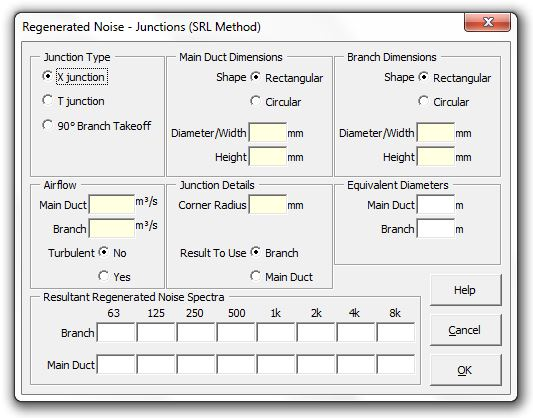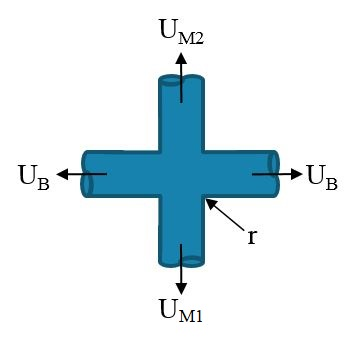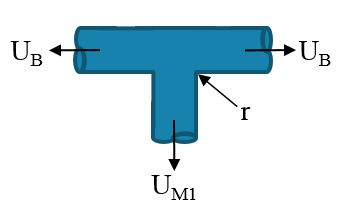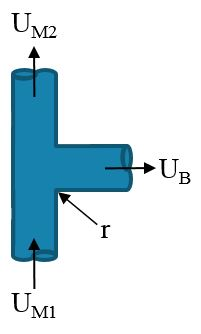### Strutt Help

Regenerated Noise - Junctions (SRL Method)    1/1

Strutt|Mechanical Services|Regenerated Noise|Junction/Split inserts sound power levels into the active row of the worksheet.Input required is the junction type, duct dimensions (main and branch) and flow rates.

SRL calculates regenerated noise from junctions using:

X-Junction frequency sound power levels are calculated by:L_(W_(branch))(f_0) = K_J + 10log_10(f_0) + 50log_10(U_B) + 10log_10(S_B D_B) - 18

Where:
f_0 is the octave band centre frequency, Hz
D_B is the equivalent diameter of the branch duct, m
U_B is the flow velocity in the branch duct, m/s
S_B is the cross sectional area of the branch duct, m2
K_J is the characteristic spectrum (taken from Figure 8.12 SRL)

and,

L_(W_(mai n))(f_0) = L_(W_(branch)) + 20log_10(D_M/D_B)

Where:
D_M is the equivalent diameter of main duct, m
D_B is the equivalent diameter of branch duct, m

T-Junction
frequency sound power levels are calculated by:L_(W_(branch))(f_0) = K_J + 10log_10(f_0) + 50log_10(U_B) + 10log_10(S_B D_B) - 18

Where:
f_0 is the octave band centre frequency, Hz
D_B is the equivalent diameter of the branch duct, m
U_B is the flow velocity in the branch duct, m/s
S_B is the cross sectional area of the branch duct, m2
K_J is the characteristic spectrum (taken from Figure 8.12 SRL for U_(M1)/U_B = 1)

and,

L_(W_(mai n))(f_0) = L_(W_(branch)) + 3

90° Branch Take Off
frequency sound power levels are calculated by:L_(W_(branch))(f_0) = K_J + 10log_10(f_0) + 50log_10(U_B) + 10log_10(S_B D_B) - 18

Where:
f_0 is the octave band centre frequency, Hz
D_B is the equivalent diameter of the branch duct, m
U_B is the flow velocity in the branch duct, m/s
S_B is the cross sectional area of the branch duct, m2
K_J is the characteristic spectrum (taken from Figure 8.12 SRL for U_(M1)/U_B = 1)

and,

L_(W_(mai n))(f_0) = L_(W_(branch)) + 20log_10(D_M/D_B)

Where:
D_M is the equivalent diameter of main duct, m
D_B is the equivalent diameter of branch duct, m

References:

• Woods Practical Guide to Noise Control, p101.
• SRL Noise Control in Building Services, pp238-240